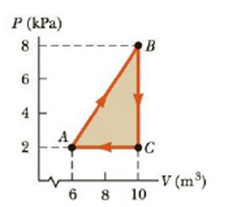Chapter 12, Problem 28P

Chapter
Section
Textbook Problem

Consider the cyclic process described by Figure P12.28. If Q is negative for the process BC and ΔU is negative for the process CA, determine the signs of Q, W, and ΔU associated with each process.Figure P12.28

(a)

To determine
The signs of Q, W and ΔU associated with the process BC

Explanation

Given Info:

The energy transferred as heat ( QBC ) is negative.

The volume is constant in the process BC,

Formula to calculate the work done of the gas is,

F=PΔV

• P is the pressure of the gas
• ΔV is the change in volume of the gas

Since, the volume is constant for the process BC; the work done on the gas (WBC) is zero.

From First law of thermodynamics,

ΔUBC=QBC+WBC <

(b)

To determine
The signs of Q, W and ΔU associated with the process CA

(c)

To determine
The signs of Q, W and ΔU associated with the process AB

Still sussing out bartleby?

Check out a sample textbook solution.

See a sample solution

The Solution to Your Study Problems

Bartleby provides explanations to thousands of textbook problems written by our experts, many with advanced degrees!

Get Started

Too little in the diet is associated with osteoporosis. a. vitamin B12 b. protein c. sodium d. niacin

Nutrition: Concepts and Controversies - Standalone book (MindTap Course List)

Name the following compounds. a Sn3(PO4)2 b NH4NO2 c Mg(OH)2 d NiSO3

General Chemistry - Standalone book (MindTap Course List)

What is an antimicrobial?

General, Organic, and Biological Chemistry